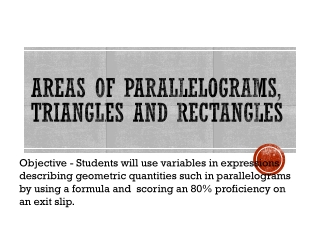DownloadDownload PresentationAreas of Parallelograms, Triangles and Rectangles

# Areas of Parallelograms, Triangles and Rectangles

Download Presentation## Areas of Parallelograms, Triangles and Rectangles

- - - - - - - - - - - - - - - - - - - - - - - - - - - E N D - - - - - - - - - - - - - - - - - - - - - - - - - - -
##### Presentation Transcript

1. Areas of Parallelograms, Triangles and Rectangles Objective - Students will use variables in expressions describing geometric quantities such in parallelograms by using a formula and scoring an 80% proficiency on an exit slip.

2. Formula for Area of Rectangle • Area = Length X Width 6cm w A= l w 10cm A=10cm x 6cm l A=60cm 2

3. Parallelograms • A parallelogram is a quadrilateral where the opposite sides are congruent and parallel. • A rectangle is a type of parallelogram, but we often see parallelograms that are not rectangles (parallelograms without right angles).

4. Area of a Parallelogram • Any side of a parallelogram can be considered a base. The height of a parallelogram is the perpendicular distance between opposite bases. • The area formula is A=bh A=bh A=5(3) A=15m2

5. Area of a Parallelogram • If a parallelogram has an area of A square units, a base of b units, and a height ofhunits, then A = bh. Example 2 Base = 15 cm Height = 12 cm Area = 15 cm x 12 cm Area = 180 sq. cm

6. Ex. 3 Find the missing unit A= base x Height A = bh • Area= 56 cm squared • Base= _______ • Height= 8 cm

7. Area of Rectangles and Triangles Objective: Students will use variables in expressions describing geometric quantities for Areas by using formulas and scoring an 80% proficiency on an exit slip.

8. Area of a Triangle • A triangle is a three sided polygon. • Any side can be the base of the triangle. • The height of the triangle is the perpendicular length from a vertex to the opposite base. • A triangle (which can be formed by splitting a parallelogram in half) has a similar area formula: A = ½ bh.

9. Example1 A= ½ bh A= ½ (30)(10) A= ½ (300) A= 150 km2

10. Example 2 14.6 cm 18.7 cm A= ½ bh A= ½ A= ½ A=

11. Triangle Vocabulary Copy this down on your graphic organizer!

12. Vocabulary Review • Right Angle - 90˚ or perpendicular • Obtuse Angle – any triangle with one angle > 90˚ • Acute Angle – any triangle with one angle < 90˚ • Equilateral Triangle – all sides of the triangle are the same length • Isosceles Triangle – 2 sides of the triangle are same length • Scalene Triangle – No sides of the triangle are the same length • Congruent – The same thing • Congruent shapes can be the same but in a different orientation (direction)

13. A = l w • A = s² • A = b h • A = ½ b h or b h 2 Formulas to Know! Add these to your graphic organizer!

14. Complex Figures • Step 1: Find what shapes make up the complex figure • Step 2: Make a grocery list of what you know • Step 3: Use the appropriate formula to find the area of each shape that makes up the whole. • Step 4: Add the areas together for the total area. • If there is another part to the question, use your critical thinking skills to determine how to get the answer

15. Example 24 cm 10 cm | 27 cm | Step 1: Split the shape into a rectangle and triangle. Step 2: The rectangle is 24cm long and 10 cm wide. The triangle has a base of 3 cm and a height of 10 cm. l= 24cm w= 10cm b=(27cm – 24cm) 3cm h=10cm

16. Step 3 & 4 Rectangle Triangle A = ½ bh A = lw A = ½ (3)(10) A = 24(10) A = 240 cm2 A = ½ (30) A = 15 cm2 Total Figure A = A1 + A2 A = 240 + 15 = 255 cm2

17. Draw and Do this in your notebook! 20 cm 5 cm | 26 cm |

18. Solution Rectangle Triangle A = ½ bh A = lw Total Figure A = A1 + A2 A =

19. work Time Textbook Questions Page 145-147 #2, 5, 9, 11 Homework: Lesson 4.4 Page 87-89 (In H/W and Practice Book)